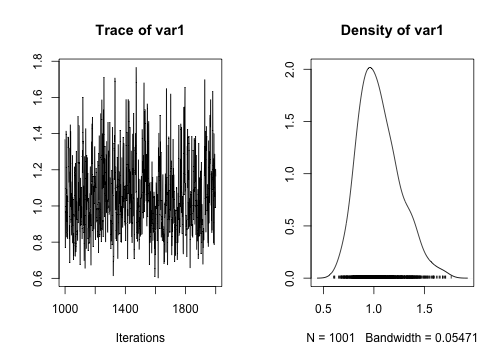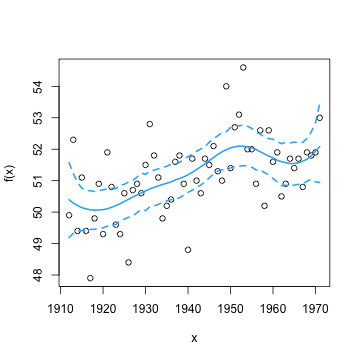## Introduction

Bayesian trend filtering estiamtes the function $$f = f(x_1), \ldots, f(x_n)$$ from data $$y_i, i=1, \ldots, n$$, hypothesized to be generated from the model $y_i = f(x_i) + \epsilon_i$ by fitting a fully Bayesian hierarchical model that is equivalent to the minimization problem $argmin_f ||y - f||_2^2 + \lambda||D^k f||_1$ as detailed by R.J. Tibshirani [-@Tibshirani:2014]. The penalty matrix $$D^k$$ estimates $k$th derivatives of $$f$$ at various inputs $$x_i$$. This is the Bayesian analogue to the function genlasso::trendfilter.

The main function of this package, btf::btf, assumes the inputs $$x_i, i=1, \ldots, n$$, are equally spaced and in ascending order. The user needs to specify the order of the derivatives $$k$$. I recommend $$k<=3$$ since calculations with $$D^k$$ can otherwise cause numerical instability. Note that unlike genlasso::trendfilter Bayesian trend filtering will not set any elements of the penalty vector $$D^k f$$ exactly equal to zero and thus this method should be used as a smoother.

## Fitting btf

We'll use the time-series observations datasets::nhtemp. First, fit a model by specifying the observations $$y$$, the order of the derivative to use, and possibly the number of iterations.

library(btf)
fit <- btf(nhtemp, k=2, iter=2000)


The variable fit is nothing special, but I recommend retrieving the posterior samples with the functions btf::getPost. You can specify which parameter you want to investigate, by default we assume you are interested in the estimate of $$f$$. Output from this function is a coda::mcmc object, suitable for any of the methods specified within the coda package.

s2_sample <- getPost(fit, 's2')
plot(s2_sample)Alternatively, you can ask for a summary of the parameters requested. The function btf::getPostEst will give the output from coda::summary.mcmc applied to the parameters specified, or $$f$$ is no paramters are specified. You can also specify a function, via the named argument est, to be applied to each of the paramters requested

getPostEst(fit, 's2', est=mean)

##     var1
## 1.054152


A simple plot of the estimate of $$f$$ and highest posterior density intervals is provided by the S3 method btf::plot.btf

plot(fit)